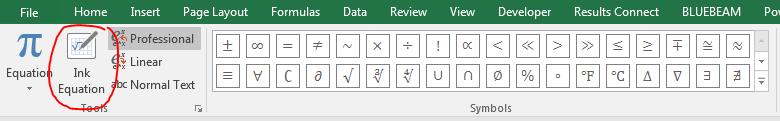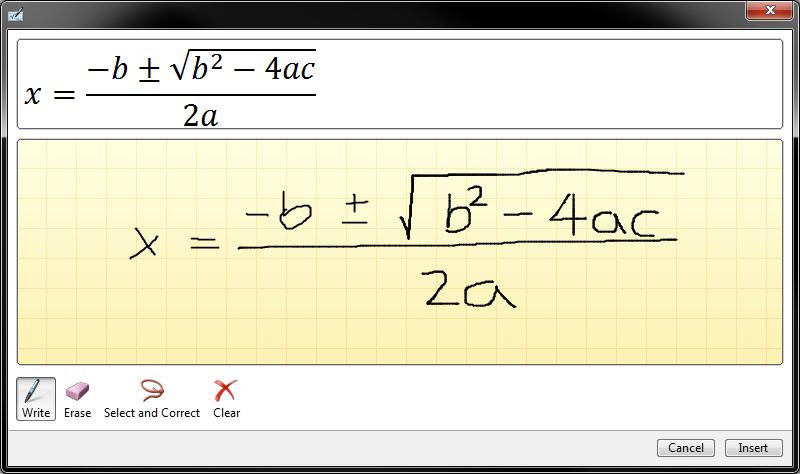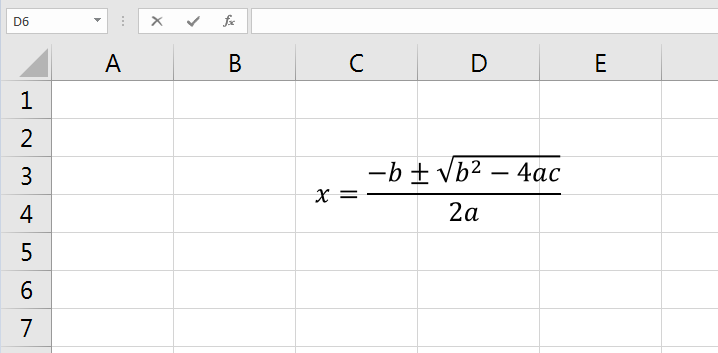# Excel Equation Editor

### Excel Equation Editor

One of Excel feature is inserting neatly formatted mathematical equation as a graphical object. Please note that these doesn't perform calculations but are used mainly for presentation.

Description of Example
There are ways to use the equation editor and we find "Ink Equation Tool" is the easiest way to do this. This tool can be accessed by Insert Tab > Equation > Design, it is under the "Tools" group. Once activated, a user can sketch or draw the equation and amazingly recognized by this tool.
This examples shows how the quadratic equation has been written by hand, and Excel automatically converts it to a nicely formatted equation.Excelcrib was founded in November 2017 by Garry, a Microsoft® Office Excel® (MS Excel) enthusiast with background in engineering. He's been using MS Excel for more than 15 years in practice with specialty in VBA.